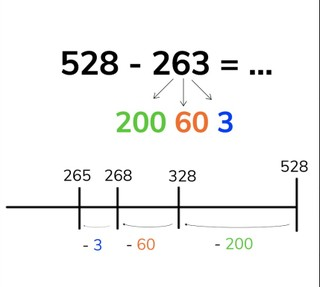Subtraction to 1,000 splitting the subtrahend with regrouping

# Subtraction to 1,000 splitting the subtrahend with regrouping

No account needed.8,000 schools use Gynzy92,000 teachers use Gynzy1,600,000 students use Gynzy

## General

Students learn to subtract numbers to 1,000 in which they split the subtrahend

2.NBT.B.7

## Relevance

Discuss with students that it is important to be able to subtract by splitting the subtrahend to make subtracting larger numbers easier.

## Introduction

Show a subtraction problem and ask students to find the difference. Ask the students to explain their strategy. Then show students a story problem and ask students to say or write the problem. You may ask students to solve individually or to solve as a class.

## Development

First explain to students that when splitting the subtrahend, you always split the subtrahend (the second number) into hundreds, tens, and ones and take them away separately from the first number (the minuend). Remind students that hundreds are a group of one hundred and end with zeros in the tens and ones places, that tens are a group of ten with a zero in the ones place, and that ones are the numbers from 1 to 9. Next explain to students that you can also subtract by splitting the subtrahend using the number line. The first number of the subtraction problem (minuend) goes at the end of the number line. Discuss that you can take jumps to take away the second number (the subtrahend). Show this to the students splitting the subtrahend into hundreds, tens and ones and jumping that on the number line. Ask students which number you jumped to on the number line. That number is your difference. Remind students that they can choose to further split a number to help cross hundreds or tens. You can split 60 into 20 and 40. That means that you first jump to 308, which makes it easier to take away the other 40. Do a few problems with the students.Then explain to students that you can also subtract splitting the subtrahend without using a number line by splitting the subtrahend into hundreds, tens, and ones, and then taking them away from the first number in steps. You first take away the hundreds. You then take the tens away from the difference you found. Then you take the ones away from that difference to find the final difference. Remind students that they can chose to split the tens into 10 and 50. Ask students which steps they take in subtracting the given problems on the interactive whiteboard. Ask students to explain their strategy.

Check that students are able to subtract by splitting the subtrahend by asking the following questions:
- What does it mean to split the subtrahend?
- What steps do you take in subtracting by splitting?
- How do you make it easier to cross hundreds or tens?

## Guided practice

Students are first given problems to solve that make use of a number line. They must take away the subtrahend in jumps. They are then asked to solve the subtraction problems by splitting the subtrahend.

## Closing

Discuss with students that it is important to be able to split the subtrahend to make it easier to subtract larger numbers. Divide the class into groups. Two groups come to the front of the class. One student must draw jumps on the number line and the other group must solve as quickly as possible. Next show the objects on the interactive whiteboard. These objects all have price tags. Ask students calculate how expensive the objects are.

## Teaching tips

Students who have difficulty splitting the subtrahend can make use of a number line. Have them practice splitting the subtrahend into discrete jumps on the number line to show what they are taking away from the first number (the minuend). Remind them to first take away the hundreds, then the tens, and end with the ones.

### The online teaching platform for interactive whiteboards and displays in schools

• Save time building lessons

• Manage the classroom more efficiently

• Increase student engagement